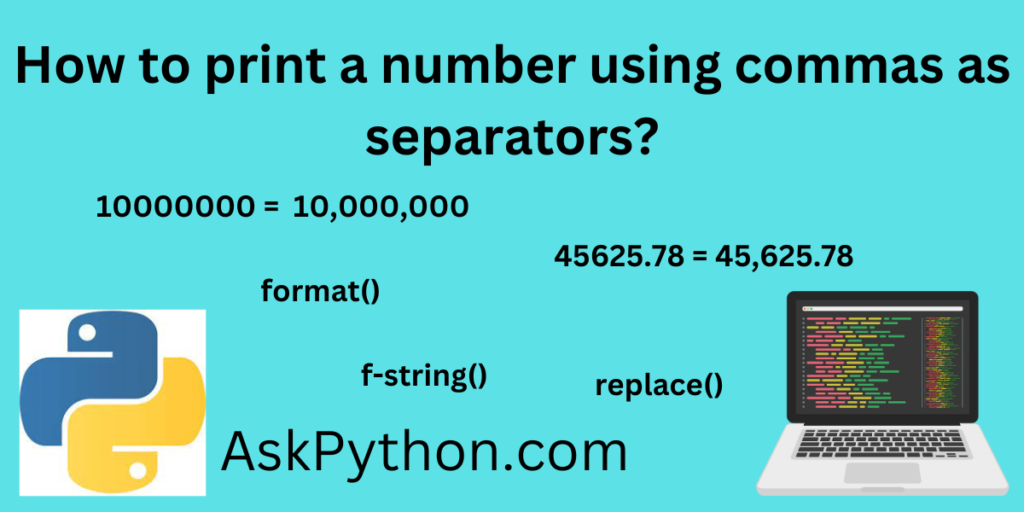# How to print a number using commas as separators?Python language combines different data types, operators, and functions. Let’s see how to print a number using commas as separators in Python.

We use integer and float data types for various mathematical operations. Python is used in different domains like machine learning and data science, Where mathematical problems are solved using different types of formulas. We use different mathematical formulas on various data types like integers and float.

## Why do we need commas as a separator in numbers?

Sometimes, we must deal with big integers and float numbers like 1000000 or 48907.6789. Visualization of these numbers is very complex compared to the smaller numbers, so we always use commas to separate them. Including the commas as a separator in this number helps us visually to recognize the number. For example, 10,00,000 is more understandable as compared to 1000000 this number.

## Integer in Python

A simple number without a fractional part or any type of number, including zero, is considered an Integer data type in Python. Integers are used in various mathematical operations. Let’s see the 1 example.

```x = 1000
print(type(x))
```

Here, the x variable is assigned with value, and the type() function is used to get the data type of value provided to the variable. The integer data type is represented as an ‘int’ in the output.

## Float in Python

A number with a floating or decimal point is considered a float data type in python. For example, 4.5, 7.89, etc.

```X= 4.5
print(type(X))
```

Here, the X variable is assigned with value, and after printing the type() function, we get the output as a float type.

## Print a Number Using Commas as Separators

### Example 1: Print Commas Using F-string Function (Integer Data Type)

The f-string is considered a ‘formatted string’ in python. The syntax of the f-string is very easy; it begins with the f letter and ends with the curly braces. The curly braces contain data that we want to replace the string. Let’s see the example of using an f-string as a commas separator.

```Number = 10000000

print(f"{Number:,d}")
```

Here, the ‘Number’ is assigned with the integer value. An f-string function is used where the variable and comma are provided as attributes of the f-string function.

### Example 2: Print Commas Using F-string and Replaces Function (Integer Data Type)

The Replace function is used to replace the values which are mentioned within curly braces. Let’s, use the replace function to add comma at the thousand place in the number.

```Number = f'{10000:,}'.replace('.' , ',')
print(Number)
```

Here, The f-string and replace functions are both used together. The ‘,’ is inserted in the provided f-string. Let’s see the output.

### Example 3: Print commas using format() function (Decimal Data Type)

The format() function is used to format the string in python. In this example, we can use the format function to add the comma at the thousand places. The format function automatically replaces a thousand places with commas. Let’s see the example with decimal number.

```Number = 456324.1234567

Result = '{:,.3f}'.format(Number)
print(Result)
```

Here, in this example 3, we have used a decimal number as input. The .format() function is used where the ‘Number’ is provided as a parameter.

### Example 4: Print commas using format() function (Integer Data Type)

The format() function is also used for the integer data types. Let’s see the implementation using integer data type.

```Result = '{:,}'.format(3456231)
print(Result)
```

Here, in this example, the result variable contains the value, which is an integer data type. the .format() function holds the integer as its attribute. Let’s see the result for the above code.